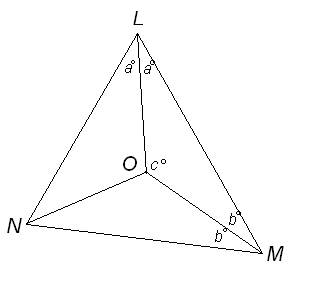#### You may also like### Building Tetrahedra

Can you make a tetrahedron whose faces all have the same perimeter?A 1 metre cube has one face on the ground and one face against a wall. A 4 metre ladder leans against the wall and just touches the cube. How high is the top of the ladder above the ground?Four rods are hinged at their ends to form a convex quadrilateral. Investigate the different shapes that the quadrilateral can take. Be patient this problem may be slow to load.

# Incentre Angle

##### Age 14 to 16 Short Challenge Level:Let
$\angle OLM = \angle OLN = a^{\circ},$
$\angle OML = \angle OMN = b^{\circ}$  and
$\angle LOM = c^{\circ}$

Angles in a triangle add up to $180^{\circ}$, so from $\triangle LMN$, $$2a^{\circ}+2b^{\circ}+68^{\circ} = 180^{\circ}$$ which gives $$2(a^{\circ}+b^{\circ})=112^{\circ}$$ In other words $$a^{\circ}+b^{\circ}=56^{\circ}$$

Also, from $\triangle LOM$, $$a^{\circ}+b^{\circ}+c^{\circ}=180^{\circ}$$ and so
\eqalign{ c^{\circ}&= 180^{\circ} - (a^{\circ}+b^{\circ})\cr &= 180^{\circ}-56^{\circ}\cr &=124^{\circ}}

This problem is taken from the UKMT Mathematical Challenges.
You can find more short problems, arranged by curriculum topic, in our short problems collection.